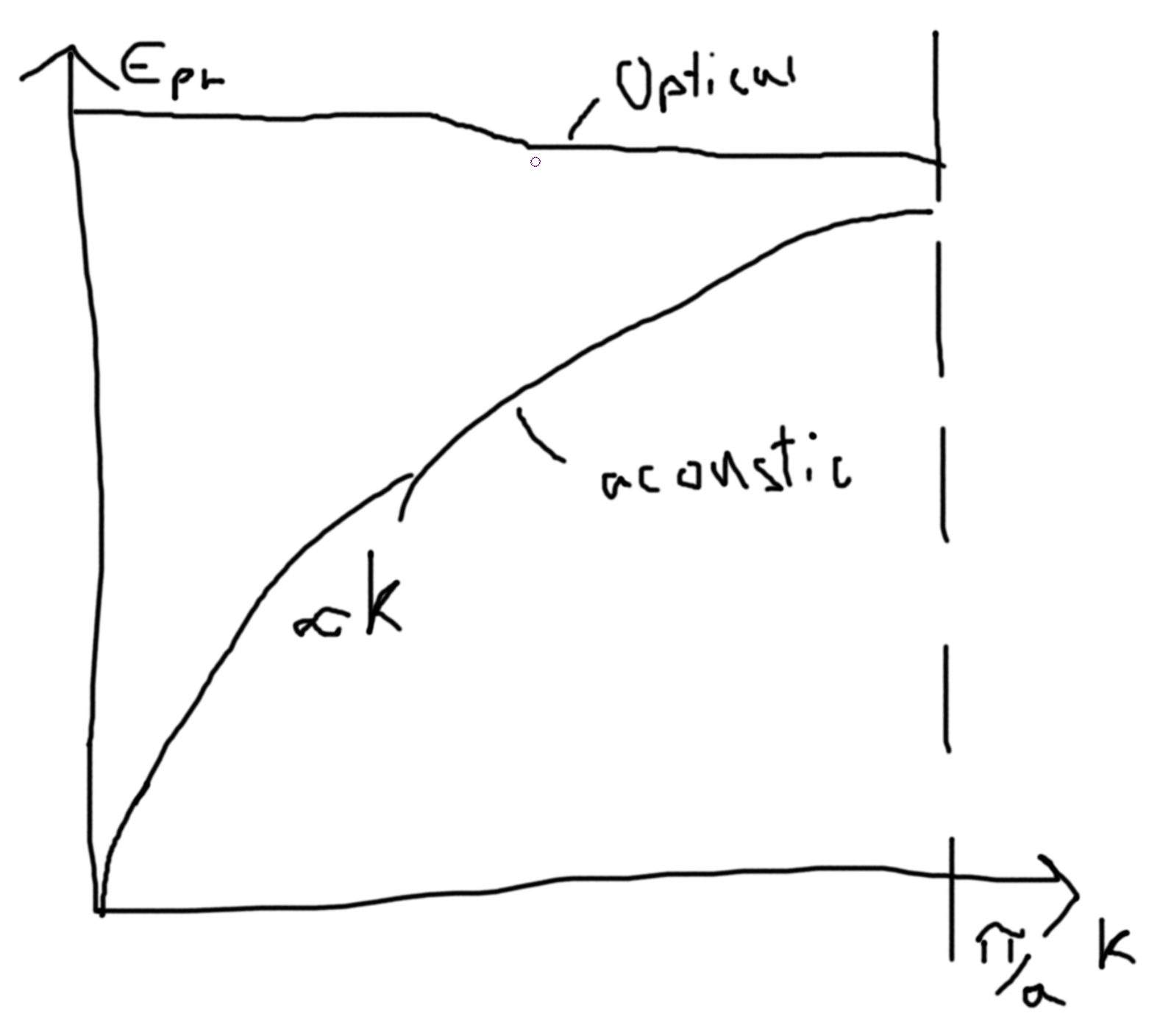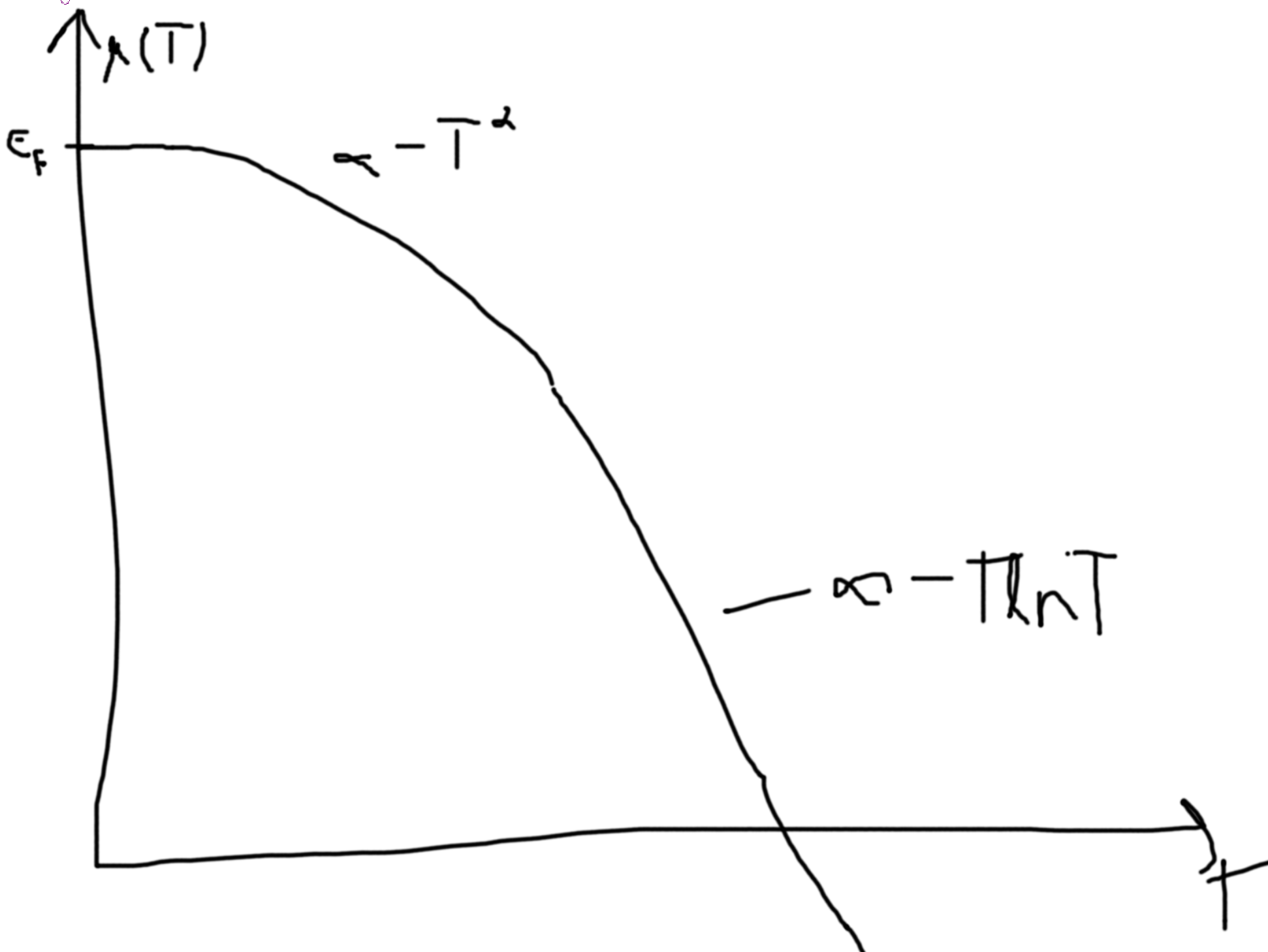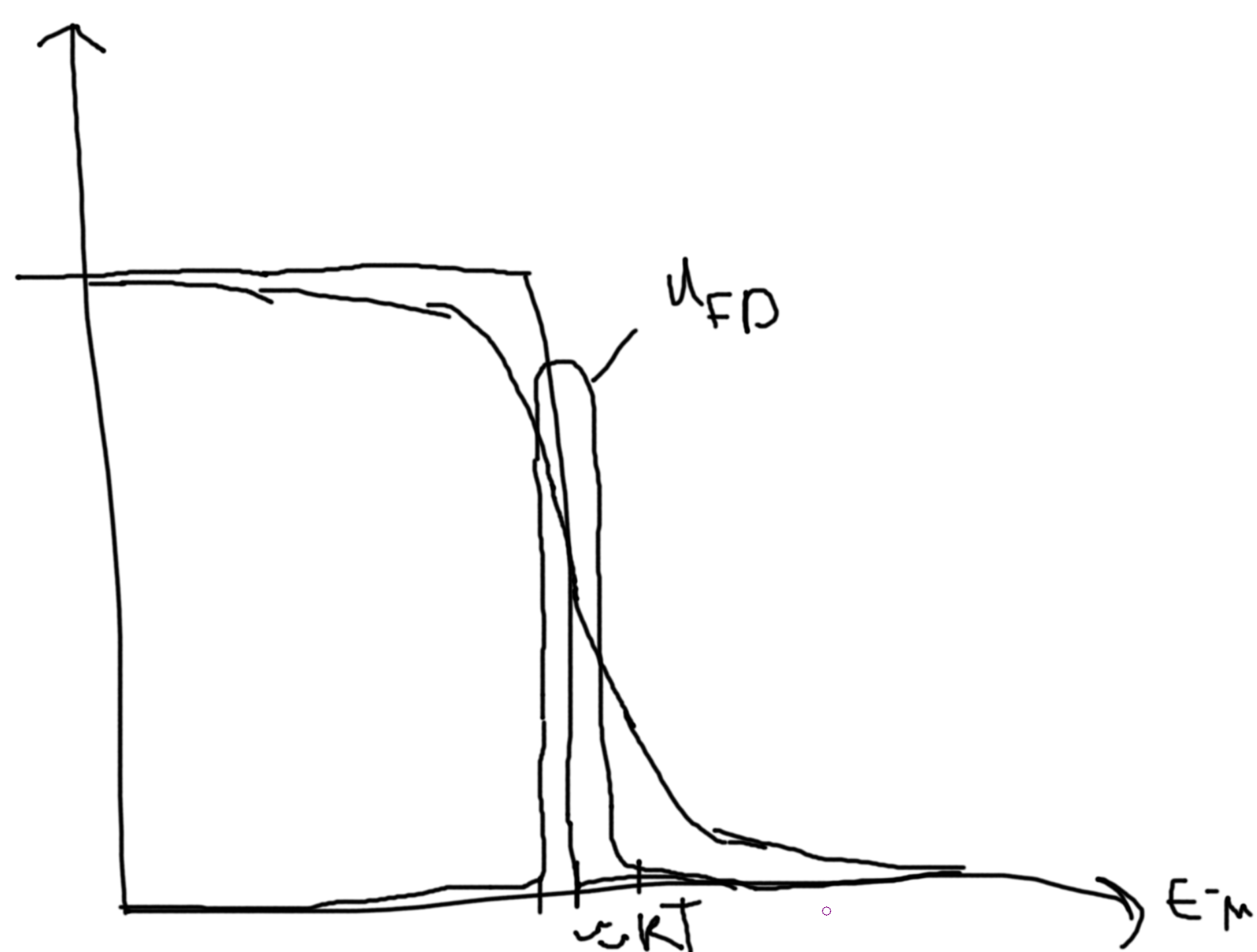# Quantum Statistical Mechanics

## QMHO

$$\hat{H} = \frac{\hat{p}^2}{2m} + \frac{m\omega^2 x^2}{2} = \hbar\omega(b^\dagger b+1/2)$$ with energies $$E_n = \left(n+\frac{1}{2}\right)\hbar\omega$$.

$$Z_{QHO} = \sum_n \exp(-\beta E_n)$$.

$$\langle E\rangle_{QHO} = \hbar\omega\left(\frac{1}{2} + \frac{1}{\exp(\beta\hbar\omega) - 1}\right)$$ with $$\langle n\rangle = \frac{1}{\exp(\beta\hbar\omega)-1}$$ - which looks like $$\langle n\rangle_{BE}$$ with $$\mu=0$$. BE is Bose-Einstein, $$E(k) = \frac{\hbar^2k^2}{2m}$$. We set $$\mu=0$$ since we can add or remove particles freely to this bosonic state without affecting the other particles.

Consider if we have an energy of $$\hbar\omega$$ and we populate it with $$n$$ particles, then we get the same $$\hat{H} = \hbar\omega(b^\dagger b + 1/2) = \hbar\omega(n+1/2)$$. Thus, we can consider a $$n-$$ energy level a bosonic gas of $$n$$ particles with energy $$\hbar\omega$$.

## Maxwell Boltazmann Quantum Statistics

$$Z_N^{MB} = \frac{1}{N!}Z_{N}^{distinguishable}$$

$$Z_1 = \sum_k \exp(-\beta E_k)$$. $$Z_N^{Non-Interacgint MB} = \frac{1}{N!}Z_1^N$$. Let $$k=1,2,3$$. $$Z_2^{NI,MB} = \frac{1}{2!}\left(\exp(-\beta E_1) + \exp(-\beta E_2) + \exp(-\beta E_3)\right)^2 = \frac{1}{2}\left(\exp(-2\beta E_1) + \exp(-2\beta E_2) + \exp(-2\beta E_3)\right) + \exp(-\beta(E_1+E_2)) + \exp(-\beta(E_2+E_3)) + \exp(-\beta(E_1+E_3))$$.

To fix partial occupancies, can take $$\frac{1}{2}\to 1$$ then $$Z_2^{NI Bosons}$$ or take $$\frac{1}{2}\to 0$$ then $$Z_2^{NI Fermions}$$.

$$Z_G^{NI,MB} = \sum_M Z_M^{NI,MB}\exp(MB\mu) = \sum_M \frac{1}{M!}\left(\sum_k \exp(-\beta E_k)\right)^M(\exp(\beta \mu))^M = \sum_M \frac{1}{M!}\left(\sum_k \exp(-\beta(E_k-\mu))\right)^M = \exp\left(\sum_k\exp(-\beta(E_k-\mu))\right) = \prod_k\exp(-\beta(E_k-\mu))$$.

$$\phi^{NI,MB} = -kT\ln Z_G^{NI,MB} = \sum_k \phi_k$$. $$\phi_k = -k_BT\exp(-\beta(E_k-\mu))$$, $$\langle n\rangle_{MB} = -\frac{\partial \phi_k}{\partial\mu} = \exp\left(-\beta(E_k-\mu)\right)$$.

So, $$\langle n\rangle = \frac{1}{\exp(\beta(E-\mu)) + \gamma},\gamma=\begin{cases}+1 & FD \\ -1 & BE \\ 0 & MB\end{cases}$$.

### Plotting Behavior of E-mu

So, for MB this looks like a decaying exponential when plotted as a function of $$E-\mu$$. For BE, there is asymototic behavior at 0 but decays above the MB exponential as $$E-\mu$$ increases. For FD, there it is half at zero and decays strictly below the MB exponeitial, at $$-\infty$$ it goes to 1.

At the classical limit, these different statistics converge to each other.

For very small $$T$$, the BE curve approaches a delta function at $$0$$. Since BE bounds the others above, the others become zero across the positive domain. The FD curve becomes a step-down function from $$1$$ to $$0$$.

### Plotting Behavior of E

The plots are shifted over by $$\mu$$. It gets more complicated if $$\mu$$ is a function of temperature. Thus, the plots shift a bit left of $$E=\mu$$ for a fixed value of $$\mu$$.

## Classical Limits

$$\langle O\rangle = \sum_\alpha p_\alpha O_\alpha, p_\alpha = \frac{1}{Z}\exp(-\beta E_\alpha)$$.

Quantum: $$\langle O\rangle = \sum_k n(E_k-\mu)O_k$$. $$N = \langle N\rangle = \sum_k n(E_k-\mu)\to N(\mu) \to \mu$$.

For Fermions at $$T=0$$, $$N = \sum_k 1\to E_k^{max,occupied} = \mu\equiv E_F=\epsilon_F$$, the Fermi energy.

Einstein Gas: basic energy is $$\varepsilon = \hbar\omega$$ the constant, and the linear model is $$\varepsilon=\hbar \gamma k$$. These give the optical phonons which are the approximately the constant and the acoustic are approximately the linear.

$$\varepsilon(k) = \frac{\hbar^2k^2}{2m}$$.

## New Term

### Review

• BE and FD statistics
• $$\langle n\rangle_{B/F} = \frac{1}{\exp(\beta(\epsilon-\mu))\mp1}$$. Recall, if $$\epsilon=\mu$$ then $$\langle n\rangle_F = \frac{1}{2}$$ and $$\langle n\rangle_B \to\infty$$.

Classical: $$\langle E\rangle = \sum_\alpha p_\alpha E_\alpha$$, $$p_\alpha = \frac{\exp(-\beta E_\alpha)}{Z}$$. For a classical system, $$Z = \frac{1}{N!}Z_1^N$$. For a quantum system, we can compute $$\langle E_1\rangle$$ to then get $$\langle E\rangle = N\langle E_1\rangle$$. I.e. for a carbon atom, since the electrons are dependent we can describe it in the classical way, but if they were independent then we would need FD or BE.

$$\langle E\rangle = \sum_{\vec{k}} = n(\epsilon(\vec{k}),T,\mu(T))\epsilon(\vec{k})$$. Need: $$\mu(T)$$. For $$N$$ quantum particles, we have $$N = N(\mu) = \sum_\alpha n_\alpha = \sum_{\vec{k}}n(\epsilon(\vec{k}),T,\mu(T))$$. We can then invert this to get $$\mu(T)$$.

## QHO

We can solve this classically since we have just one particle (i.e. one oscillator). $$E_n = \left(n + \frac{1}{2}\right)\hbar\omega$$. $$\langle E\rangle = -\frac{\partial\ln Z}{\partial\beta}$$. $$Z = \sum_n \exp(-\beta E_n) = \exp(-\beta\hbar\omega/2)\frac{1}{1-\exp(-\beta\hbar\omega)}$$. So, $$\langle E\rangle = \hbar\omega\left(\frac{1}{2} + \frac{1}{\exp(\beta\hbar\omega)-1}\right) = \left(\langle n\rangle+\frac{1}{2}\right)\hbar\omega$$.

## Density of States

$$N(,\mu,T) = \sum_k\langle n(\epsilon_k,\mu,T)\rangle\delta(\epsilon-\epsilon_k) = \int n(\epsilon(\vec{k}),\mu,T)g(\epsilon)d\epsilon$$.

### Particles in a Box

$$V = L^D$$, for $$D-$$ dimensions.

You can do this with:

1. Standing Waves

$$\Psi\propto \sin\left(\frac{\pi}{L}n_xx\right)$$, $$n\in\mathbb{Z}^+$$. For the dimensions we have, each state takes up a volume of $$\left(\frac{L}{\pi}\right)^D$$. Note, we only integrate over one quadrant, hence $$1/2^D$$ of the total space $$\to \left(\frac{L}{2\pi}\right)^D$$.

1. Plane Waves (Periodic Boundary Conditions)

So, $$\Psi = \frac{1}{\sqrt{V}}\exp(i\vec{k}\cdot\vec{r})$$. Our $$k$$ is then, $$\frac{2\pi}{L}(n_x,n_y,n_z)^T$$. Then, $$n_i\in\mathbb{Z} = \{0,\pm1,\pm2,\cdots\}$$. For the dimensions we have, each state takes up a volume of $$\left(\frac{L}{2\pi}\right)^D$$.

We need $$\epsilon(\vec{k})$$.

1. For massive fermions, $$\epsilon(\vec{k}) = \frac{\hbar^2k^2}{2m}$$, from solving the Scrodinger Equation.
2. For massive bosons, $$\epsilon(\vec{k}) = c\cdot\vec{p} = c\hbar|\vec{k}| = c\hbar k$$.

If $$\epsilon(\vec{k})\propto f(|\vec{k}|)$$. $$g(\epsilon)d\epsilon = 4\pi k^2\sum_{k_i}\left|\frac{dk_i}{d\epsilon}\right|^2d\epsilon \frac{V}{(2\pi)^3}g(k)$$.

We use the simpler form, $$g(\epsilon)d\epsilon = 4\pi k^2\left|\frac{dk}{d\epsilon}\right|^2d\epsilon \frac{V}{(2\pi)^3}g(k)$$ For (1), $$k^2 = \frac{2m\epsilon}{\hbar^2}$$, $$\frac{dk}{d\epsilon} = \frac{1}{2}\sqrt{\frac{2m}{\hbar^2\epsilon}}$$. Then, $$g(\epsilon)d\epsilon = \frac{V}{4\pi^2}\left(\frac{2m}{\hbar^2}\right)^{3/2}\sqrt{\epsilon}d\epsilon$$.

Note, we could also write, $$g(\epsilon) = \frac{1}{V}\sum_{\vec{k}}\delta(\epsilon-\epsilon_{\vec{k}}) = \frac{1}{L^3}\frac{1}{\left(\frac{2\pi}{L}\right)^D}\int d\vec{k}\delta(\epsilon-\epsilon_{\vec{k}}) = \frac{1}{(2\pi)^D}\int d\vec{k}\delta(\epsilon-\epsilon_{\vec{k}}) = \begin{cases} 4\pi \int dk k^2 & D=3 \\ 2\pi\int dk k^1& D=2\\2\int dk k^0&D=1\end{cases}$$. Then, $$k\to \epsilon$$. $$d\epsilon = \left|\frac{d\epsilon}{dk}\right|dk$$.

For phonons, $$g(k) = 2$$, so it is typically not written.

$$\omega = ck$$. $$c = \nu\cdot\lambda = \frac{\omega}{2\pi}\frac{2\pi}{k}$$. $$\epsilon_{ph} = \hbar ck = \hbar\omega$$.

Consider a box with a small hole in it. Put a photonic gas (Electromagnetic Radiation) in the box at a temperature $$T$$.

$$g(\omega)d\omega = 2\frac{V}{(2\pi)^3}4\pi k^2\frac{dk}{d\omega}d\omega$$ Note the leading two comes from $$g(k) = 2$$ for the photonic gas. Then, $$k^2 = \frac{\omega^2}{c^2}$$. $$\frac{dk}{d\omega}=\frac{1}{c}$$.

Then, $$g(\omega)d\omega = \frac{V\omega^2}{\pi c^3}d\omega$$.

The energy density is then, $$U(\omega)d\omega = V u(\omega)d\omega = \epsilon(\omega)g(\omega)n_{ph}(\omega,T)d\omega$$. $$n_{ph}(\omega,T) = n_B(\mu=0)$$.

$$U(\omega)d\omega = Vu(\omega)d\omega = \frac{\hbar\omega g(\omega)}{\exp(\beta\hbar\omega)-1}d\omega = \frac{V\hbar \omega^3}{\pi^2 c^3}\frac{1}{\exp(\beta\hbar\omega)-1}d\omega$$. So, $$u(\omega) = \frac{\hbar \omega^3}{\pi^2 c^3}\frac{1}{\exp(\beta\hbar\omega)-1}$$.

### Aside

Remember that $$n_F = \frac{1}{\exp\left(\beta(\epsilon(k)-\mu)\right)-1}$$.

## Lecture 2

Consider that we have energy levels $$\epsilon_i$$ for each particle. So, classically, $$\langle\epsilon\rangle = \sum p_n\epsilon_n$$ and $$\langle E\rangle = N\langle\epsilon\rangle = \sum Np_n\epsilon_n = \sum n\epsilon_n$$. Quantum mechanically, $$\langle\epsilon\rangle = \sum_k n^{FD/BE}(\epsilon_k,\mu,T)\epsilon_k$$.

Remember for fermions, if there is a particle in $$\epsilon_1$$ then there are no other particles in $$\epsilon_1$$.

### Other Boson Systems

Lattice vibrations in solids (phonons).2 Models:

• Einstein model $$\epsilon_{ph} = \hbar\omega_0 = const$$. We did this microcanonically in HW 641. $$U(T) = 3N_A\frac{\hbar\omega_0}{\exp\left(\frac{\hbar\omega}{kT}\right)-1}$$. Low temp, $$C_V\propto\exp\left(-\frac{\hbar\omega_0}{kT}\right)$$.
• Debye Model $$\omega(k) = v_Sk$$. Where $$v_S = \frac{\partial \omega}{\partial k}$$ is the speed of sound. That makes the acoustic curve linear. Has to fix $$N$$ for the 3N modes. Find $$\omega_{max}$$ from fixing $$N$$ phonons which is $$3N_A$$ times number of atoms. For low temperature, $$C_V(T)\propto T^3$$ which is correct and Einstein’s model has the wrong prediction.

### Fermions

They obey Fermi-Dirac statistics.

$$u_{FD} = \frac{1}{\exp(\beta(\epsilon-\mu))+1}; \epsilon(k) = \frac{\hbar^2k^2}{2m}$$. The density of states is then $$g(\epsilon) = \frac{1}{4\pi^2}\left(\frac{2m}{\hbar^2}\right)^{3/2}\sqrt{\epsilon}$$ and $$N = \int d\epsilon u_{FD}(\epsilon,\mu,T)g(\epsilon)$$.

Can’t solve in general, so we do it in 3 stages.

1. $$T=0$$ (HW)
2. High $$T$$
3. Low $$T$$

For $$T=0$$. $$u_{FD} = \Theta(x)$$. $$\mu(T=0) = \epsilon_F$$. So we want $$N = \int d\epsilon \Theta(\mu(T=0) - \epsilon)Vg(\epsilon)$$. Then, $$=\int_0^{\epsilon_F}d\epsilon (2)\frac{V}{4\pi^2}\left(\frac{2m}{\hbar^2}\right)^{3/2}\sqrt{\epsilon}$$. The two comes from spin 1/2. $$n = \frac{N}{V} = \cdots = \frac{1}{3\pi^2}\left(\frac{2m}{\hbar^2}\right)^{3/2}\epsilon_F^{3/2}$$. Thus, $$\epsilon_F = \left(3\pi n\right)^{2/3}\frac{\hbar^2}{2m}$$.

$$N = \sum_{\vec{k},\sigma} n_{FD}(T=0,\epsilon_{k}) = 2\frac{V}{(2\pi)^3}\int dk\Theta(\epsilon_F-\epsilon)$$. $$\Theta(\epsilon_F-\epsilon)\to\Theta(k_F-k)$$ from $$\epsilon_F = \frac{\hbar^2 k_F^2}{2m}$$.

For high $$T$$. Recall for an ideal gas: $$F = NkT\left(\ln\frac{N}{V}\lambda^3-1\right)$$ eq [6.24]. $$\mu = \left.\frac{\partial F}{\partial N}\right|_{T,V} = \cdots = kT\ln(n\lambda^3)$$. Then, for our case, $$u_{FD} = \frac{1}{\exp(\beta(\epsilon-\mu))+1}\to_{\mu < \epsilon} \exp(-\beta(\epsilon-\mu))$$. So, $$N/V = n = \int d\epsilon \exp(-\beta(\epsilon-\mu))2\frac{1}{4\pi^2}\left(\frac{2m}{\hbar^2}\right)^{3/2}\sqrt{\epsilon} = \cdots = \left(\frac{2m}{\hbar}\right)^{3/2}\sqrt{\pi}(kT)^3\exp(\mu/kT)$$. Solving this for $$\mu$$ gives you $$\mu = kT\ln(n\lambda^3)$$.

For low $$T$$. So, $$kT\ll \epsilon_F$$. The typical scale of $$\epsilon_F$$ is roughly $$4-5 eV$$. Note that $$1eV \sim 10000 K$$.

$$\frac{\phi}{V} = 2(-b(\mu)-\frac{\pi^2}{6}g(\mu)(kT)^2 + \mathcal{O}(kT)^4)$$, $$b(\mu) = \int_{-\infty}^\mu d\epsilon a(\epsilon)$$ and $$a(\epsilon) = \int_{-\infty}^\epsilon d\tilde{\epsilon}g(\tilde{\epsilon})$$.

$$Z_G = \prod_k Z_{G_k} = \prod_k \left(1+\exp\left(-\frac{\epsilon_K-\mu}{kT}\right)\right)$$, $$\phi = -kT\ln Z_G = -kT\sum_n\ln\left(1+\exp\left(-\frac{\epsilon_n-\mu}{kT}\right)\right)\to -kTV\int_{-\infty}^\infty d\epsilon g(\epsilon)\ln\left(1+\exp\left(-\frac{-\epsilon-\mu(T)}{kT}\right)\right)$$ Invert $$N = N(\mu(T))$$ to get $$\mu(T)$$. Get $$N$$ from $$N = -\left(\frac{\partial \phi}{\partial \mu}\right)_{V,T} = 2\left(\frac{\partial b}{\partial\mu} + \frac{\pi^2}{6}\frac{\partial g(\epsilon)}{\partial\epsilon}|_{\mu}(kT)^2\right)$$. $$\frac{\partial b}{\partial\mu} = a(\mu) \approx a(\epsilon_F) + (\mu - \epsilon_F)\left(\frac{\partial a}{\partial\epsilon}\right)_{\epsilon_F} = \frac{N}{2} + (\mu-\epsilon_F)g(\epsilon_F)$$. $$N = 2\left(\frac{1}{2}N + (\mu-\epsilon_F)g(\epsilon_F) + \frac{\pi^2}{6}\left(\frac{\partial g(\epsilon)}{\partial \epsilon}\right)_{\epsilon_F}(kT)^2\right)$$. Thus, the second and third term must equal zero. So, $$\mu(T) = \epsilon_F - \frac{\pi^2}{6}\frac{1}{g(\epsilon_F)}\left(\frac{\partial g}{\partial \epsilon}\right)_{\epsilon_F}(kT)^2$$.• Electrons are fermions with spin 1/2. $$2g_F = g_{e-}$$

Created: 2023-06-25 Sun 02:31

Validate